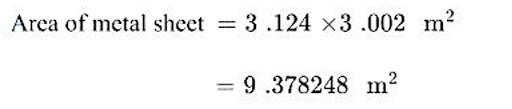# The length and breadth of a metal sheet are 3.124m and 3.002 m respectively. the area of this sheet up to the correct number of significant

more_vert

The length and breadth of a metal sheet are 3.124m and 3.002 m respectively. the area of this sheet up to the correct number of significant figure  is

A) 9.378 m2

B) 9.37 m2

C)9.378248 m2

D)9.3782 m2

more_vert

verified

Correct option is A) 9.378 m2result must have significant figures equal to least figures being multiplid do area of metal sheet 9.378 m2  (four significant digits)